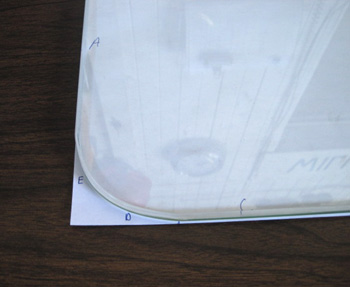# How to Figure a Radius Corner Dimension

 To figure a true quarter corner radius, use a piece of paper and run one edge along one edge of the furniture (A in the example) and the other edge along the other edge (C in the example).  Now simply mark the paper where the radius begins and measure to the corner of the paper. Double check by measuring both the A and C edges.  Also if there is more than one rounded corner measure those as well. Another very simple way, but not as accurate, is to take a dime, nickel or quarter and place it on the corner. If one of the coins matches up, then that is the radius. For instance, if a dime has the radius you need, it is called a “dime radius.”